尊敬的用户：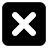• 课后作业
• 同步试卷
• 本节重点
• 主讲老师

一、选择题

1．(2016江苏扬州江都二中第一次月考)下列方程中，关于x的一元二次方程是    ()
A．x²+2x=x²-1
B．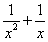-2=0
C．ax²+bx+c=0
D．3(x+1)²=2(x+1)

【试题解析】：选项A中方程可化为2x+1=0，不是一元二次方程；选项B中方程不是整式方程，不是一元二次方程；选项C中没有指明a≠0,不一定是一元二次方程；选项D中方程可化为3x²+4x+1=0．是一元二次方程．

【参考答案】：D

2．(2016广东揭阳揭西期末)若方程xᵐ⁻¹+2x-3=0是一元二次方程，则m的值为    ()
A．0
B．1
C．2
D．3

【试题解析】：根据一元二次方程的定义可得m-1=2，∴m=3．

【参考答案】：D

3．(2015新疆石河子十八中模拟)把方程x(x+2)=5(x-2)化成一般形式，则a，b，c的值分别是    ()
A．1，-3，10
B．1，7，-10
C．1，-5，12
D．1，3，2

【试题解析】：原方程可化为x²-3x+10=0，所以a，b，c的值分别是1，-3 ,10.

【参考答案】：A

4．某校准备修建一个面积为180平方米的矩形活动场地，它的长比宽多11米，设场地的宽为x米，则可列方程为    ()
A．x(x-11)=180
B. 2x+2(x-11)=180
C．x(x+11)=180
D．2x+2(x+11)=180

【试题解析】：宽为x米，则长为(x+11)米，根据题意得x(x+11)=180，故选C．

【参考答案】：C

5．(2017江苏泰兴济川中学月考)已知x=-1是一元二次方程x²+mx+n=0的一个根，则(m-n)²的值为  ()
A．0
B．1
C．2
D．4

【试题解析】：把x=-1代入方程得1-m+n=0，即m-n=1，则(m-n)²=1，故选B．

【参考答案】：B

6．方程(m+2)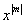+mx-8=0是关于x的一元二次方程，则()
A．m=±2
B．m=2
C．m=-2
D．m≠±2

【试题解析】：由一元二次方程的定义可得|m|=2且m+2≠0，解得m=2．

【参考答案】：B

7．(2017山东滨州期中)下列方程中，是一元二次方程的是    ()
①3x²+x=20，②2x²-3xy+4=0，③x²-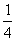=4，④x²=0，⑤x²-3x-4=0．
A．①②
B．①②④⑤
C．①③④
D．①④⑤

【试题解析】：①该方程符合一元二次方程的定义，故①是一元二次方程；②该方程中含有2个未知数，故②不是一元二次方程；③该方程是分式方程，故③不是一元二次方程；④该方程符合一元二次方程的定义，故④是一元二次方程；⑤该方程符合一元二次方程的定义，故⑤是一元二次方程，综上所述，是一元二次方程的是①④⑤，故选D．

【参考答案】：D

8．(2017江苏无锡江阴石桥中学期中)生物兴趣小组的学生，将自己收集的标本向本组其他成员各赠送一件，全组共互赠了182件，如果全组有x名同学，则根据题意列出的方程是    ()
A．x(x+1)=182
B. x(x-1)=182
C．2x(x+1)=182
D．x(x-1)=182×2

【试题解析】：全组有x名同学，每人赠送(x-1)件标本，由题意可得x(x-1)=182，故选B．

【参考答案】：B

9．(2016浙江台州中考)有x支球队参加篮球比赛，共比赛了45场，每两队之间都比赛一场，则下列方程中符合题意的是    ()
A．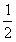x(x-1)=45
B．x(x+1)=45
C．x(x-1)=45
D．x(x+1)=45

【试题解析】：由题意，知每队与其他的(x-1)队比赛一场，则每队比赛(x-1)场，且任何两队只比赛一场，故比赛场数为x(x-1)，∴x(x-1)=45．故选A．

【参考答案】：A

10.(2013黑龙江牡丹江中考)若关于x的一元二次方程ax²+bx+5=0(a≠0)的解是x=1，则2013-a-b的值是()
A．2018
B．2008
C．2014
D．2012

【试题解析】：将x=1代入方程可得a+b+5=0,∴a+b=-5，∴2013-a-b=2013-(a+b)=2013-(-5)=2018，故选A.

【参考答案】：A

11．(2016内蒙古包头中考)若关于x的方程x²+(m+1)x+=0的一个实数根的倒数恰是它本身，则m的值是    ()
A．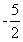B．C．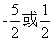D．1

【试题解析】：倒数等于它本身的数是±1，即该方程的根为x=1或x=-1．把x=1代入原方程得1+(m+1)+=0，解得m=；把x=-1代入原方程得1-(m+1)+=0，解得m=．故选C．

【参考答案】：C

12．关于x的方程ax²+bx+c=0(a≠0)中的a、b、c满足a+b+c=0和4a-2b+c=0，则方程的根分别为    ()
A．1、0
B. -2、0
C．1、-2
D．-1、2

【试题解析】：把x=1代入方程可得到a+b+c=0，把x=-2代入方程可得到4a-2b+c=0,所以方程的根分别为1、-2．故选C．

【参考答案】：C

二、填空题

1.一元二次方程(x+3)(x-3)=2x化为一般形式后，其二次项系数为，一次项系数为，常数项为

【试题解析】：整理方程得x²-2x-9=0，二次项系数为1，一次项系数为-2．常数项为-9．

【参考答案】：

1、-2、-9

2．(2014黑龙江牡丹江中考)现有一块长80 cm，宽60 cm的矩形钢片，将它的四个角各剪去一个边长为x cm的小正方形，做成一个底面积为1500 cm²的无盖的长方体盒子，根据题意列方程，化简可得

【试题解析】：根据题意，得(80-2x)(60-2x)=1500，

【参考答案】：x²-70x+825=0

3．若x=1是关于x的方程x²-px+q=0的一个根，则代数式p²-q²-2q的值是

【试题解析】：∵x=1是关于x的方程x²-px+q=0的一个根，∴1-p+q=0,∴p-q=1,∴p²-q²-2q=(p+q)(p-q)-2q=p+q-2q=p-q=1．

【参考答案】：

1

4．(2015江苏泰州泰兴二模)已知x=-1是一元二次方程ax²+bx-10=0的一个解，且a≠-b，则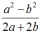的值为

【试题解析】：∵x=-1是一元二次方程ax²+bx-10=0的一个解，∴a-b-10=0，∴a-b=10．∵a≠-b，∴a+b≠0,∴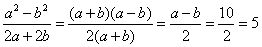【参考答案】：

5

5．一元二次方程ax²+bx+c=0的一个根是1，且(a、b、c满足b=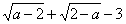，则a=，b=，c=

【试题解析】：∵b=，∴a-2≥0，2-a≥0，解得a=2，∴b=0+0-3=-3．∵一元二次方程ax²+bx+c=0的一个根是1，代入得2×1²+(-3)×1+c=0，解得c=1．

【参考答案】：

2、-3、1

6．(2016内蒙古呼伦贝尔一模)已知x=1是方程x²-3x+m=0的一个解，则m=

【试题解析】：根据题意，得1-3+m=0，即m-2=0，解得m=2．

【参考答案】：

2

7．(2016江苏泰兴洋思中学第一次质检)关于x的一元二次方程(a-1)x²+x+a²-1=0的一个根是0，则a值为

【试题解析】：把x=0代入方程可得a²-1=0，解得a=±1．又根据一元二次方程的定义可知a-1≠0，即a≠1，∴a=-1.

【参考答案】：-1

8．(2012广西柳州中考)一元二次方程3x²+2x-5=0的一次项系数是.

【试题解析】：方程3x²+2x-5=0为一般形式，2x是一次项，2是一次项系数．

【参考答案】：

2

9．(2016山东菏泽中考)已知m是关于x的方程x²-2x-3=0的一个根，则2m²-4m=.

【试题解析】：∵m是关于x的方程x²-2x-3=0的一个根,∴m²-2m-3=0,∴m²-2m=3，则2m²-4m=2(m²-2m)=2×3=6.

【参考答案】：

6

10．(2015四川绵阳中考)关于m的一元二次方程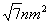-n²m-2=0的一个根为2，则n²+n⁻²=.

【试题解析】：把m=2代入原方程，得4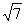n-2n²-2=0，显然n≠0，【参考答案】：

26

三、主观题

1．下列哪些数是方程x²-6x+8=0的根？
0,1,2,3,4,5,6,7,8,9,10.

【参考答案】：把0，1，2，3，4，5，6，7，8，9，10分别代入方程x²-6x+8=0中，发现当x=2或x=4时，方程x²-6x+8=0成立，所以x₁=2．x₂=4是方程x²-6x+8=0的根．

2.已知关于x的一元二次方程ax²+bx+c=0(a≠0).
(1)若a+c=-b，求证：x=1必是该方程的一个根；
(2)当a，b，c之间的关系是_________时，方程必有一根是x=-1．

【参考答案】：(1)证明：∵a+c=-b，∴a+b+c=0．

∴x=1必是方程ax²+bx+c=O(a≠0)的一个根．
(2)当x=-1时，ax²+bx+c=a×(-1)²+b×(-1)+c=a-b+c=0．
∴当a-b+c=0时，方程ax²+bx+c=0(a≠0)必有一根是x=-1．137****1878

2019-06-03 21:33:52

[北京市] 讲的好，谢谢170****1900

2019-01-16 16:38:08

[北京市] 讲的真好，赞170****0033

2018-12-11 10:40:04

[北京市] 挺好的，可以同步学习157****4488

2018-12-10 17:59:55